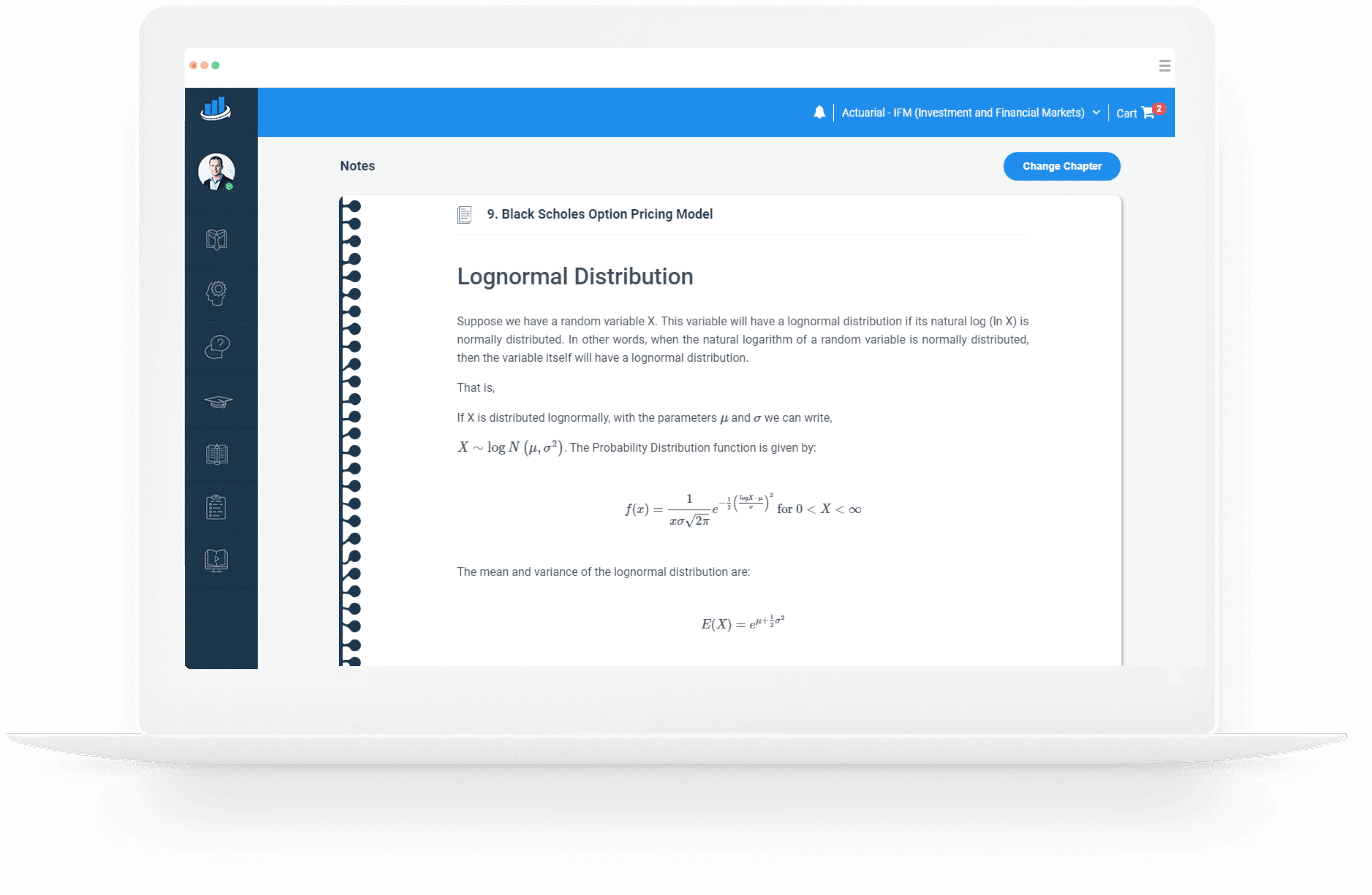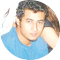Study Materials for 2023 CFA®, FRM®, Actuarial, GMAT® and EA® Exams

# SOA Exam IFM Prep Notes

### Online and Printable Prep Books for Exam IFM

Exam IFM is a three-hour multiple-choice examination designed to build your knowledge of the theoretical elements of corporate finance and financial models. Exam IFM has the most number of topics among all the three preliminary exams – a total of 10.  AnalystPrep’s team of academics and practicing actuaries has developped concise prep notes focusing all of the rudimentary aspects of financial markets.

With AnalystPrep’s concise prep notes for Exam IFM, you can simply read on your tablet, computer, or print each concept before jumping into the question bank portion of the platform.

3.5  Million
50  Thousand
# 1  Rated
###### preparation platform according to review websites## What are the Ten Topics?

Exam IFM should be taken right after having sat and passed SOA exams P and FM, since financial market concepts have the following foundations:

• Calculus and probability – part of the Exam P syllabus
• Interest theory – found in the Exam FM curriculum

Exam IFM could be further subdivided into three main themes – general finance theories (topics 1 to 3), project analysis and capital structure (topics 4 and 5), and derivatives pricing (topics 6 to 10).

 Topic Weighting 1 Mean-Variance Portfolio Theory 10-15% 2 Asset Pricing Models 5-10% 3 Market Efficiency and Behavioral Finance 5-10% 4 Investment Risk and Project Analysis 10-15% 5 Capital Structure 10% 6 Introductory Derivatives – Forwards and Futures 5-10% 7 General Properties of Options 10-15% 8 Binomial Pricing Models 10% 9 Black-Scholes Option Pricing Model 10-15% 10 Option Greeks and Risk Management 5-10%

## All of the Content on One Platform

AnalystPrep's revolutionary prep platform is an all-inclusive solution to passing exam IFM from the Society of Actuaries.

#### IFM Prep Notes

Read our study notes and learn every concept that relates to Investment and Financial Markets. Each concept is accompanied by a practical question example so you understand the calculation you will be required to perform on exam day.

#### Question Bank + Quizzes

Jump into the question bank portion of the platform and start solving as many practice questions as possible. Repetition the key in passing actuarial exams—mostly those that are calculation-intensive.## Lognormal Distribution

Suppose we have a random variable X. This variable will have a lognormal distribution if its natural log (ln X) is normally distributed. In other words, when the natural logarithm of a random variable is normally distributed, then the variable itself will have a lognormal distribution.

That is,

If X is distributed lognormally, with the parameters $$\mu$$ and $$\sigma$$ we can write,

$$X \sim \log { N\left( \mu,\sigma^2 \right) }$$. The Probability Distribution function is given by:

$$f(x)=\frac {1}{x \sigma \sqrt{2\pi }} e^{-\frac {1}{2} \left( \frac {logX-\mu}{\sigma} \right)^2 } \text { for } 0 < X < \infty$$

The mean and variance of the lognormal distribution are:

$$E(X)=e^{\mu+\frac {1}{2} \sigma^2 }$$

And

$$Var(X)=e^{2\mu+\sigma^2 }{(e^{\sigma^2 }-1)}$$

The two most important characteristics of the lognormal distribution are as follows:

• It has a lower bound of zero, i.e., a lognormal variable cannot take on negative values
• The distribution is skewed to the right, i.e., it has a long right tail.

These characteristics are in direct contrast to those of the normal distribution which is symmetrical (zero skews) and can take on both negative and positive values. As a result, the normal distribution cannot be used to model stock prices because stock prices cannot fall below zero. The lognormal distribution is also used to value options.

###The Lognormal Property of Stock Prices

Black Scholes and Merton employed a model that assumed that percentage changes in the stock price in a short period of time is normally distributed. Now, define the following:

$$\mu$$-expeted return on the stock per year

$$\sigma$$-volatility of the stock price per year

Intuitively, the return in time $$\Delta t$$ is $$\mu \Delta t$$ and the standard deviation is $$\sigma \sqrt{\Delta t}$$. This implies that:

$$\frac {\Delta S}{S}=\Phi(\mu \Delta t,\sigma^2 \Delta t)$$

where $$\Delta S$$ is the change in the stock price at time $$\Delta t$$ and $$\Phi(m,v)$$ is the normal distribution with the mean m and the variance v.

Without proof, it implies that:

\begin{align*} & ln⁡S_T-ln⁡S_0 \sim \Phi \left[ \left( \mu-\frac {\sigma^2}{2}\right)T,\sigma^2 T \right] \\ \Rightarrow &\frac {ln⁡S_T}{ln⁡S_0} \sim \Phi \left[ \left( \mu-\frac {\sigma^2}{2}\right)T,\sigma^2 T \right] \\ \end{align*}

And also

$$ln⁡S_T \sim \Phi \left[ln⁡S_0+ \left(\mu-\frac {\sigma^2}{2} \right) T,\sigma^2 T \right]$$

The last expression can be written as:

$$ln⁡S_T \sim N \left[ln⁡S_0+ \left(\mu-\frac {\sigma^2}{2} \right) T,\sigma^2 T \right]…………(1)$$

Where we will concentrate on, where:
$$S_T$$=stock price at time T
$$S_0$$=stock price at time 0
$$\mu$$=expected return on stock per year
$$\sigma$$=annual volatility of the stock price

If we let $$\delta$$ be the dividend yield then, (1) becomes:

$$ln⁡S_T \sim N \left[ln⁡S_0+ \left(\mu- \delta – \frac {\sigma^2}{2} \right) T,\sigma^2 T \right]$$

The subtraction of dividend yield is necessary since higher dividend yield means a lower future stock price.

Note: The above relationship holds because mathematically, if the natural logarithm of a random variable x(lnx) is normally distributed, then xx has a lognormal distribution. It’s also imperative to note that the BSM model assumes stock prices are lognormally distributed, with stock returns being normally distributed. Specifically, continuously compounded annual returns are normally distributed with:

a mean of $$\left[\mu-\frac {\sigma^2}{2} \right]$$ and variance of $$\frac {\sigma^2}{T}$$

Example

ABC stock has an initial price of 60, an expected annual return of 10%, and annual volatility of 15%. Calculate the mean and the variance of the distribution of the stock price in six months. 1. $$\mu$$=4.139,$$\sigma$$=0.011 2. $$\mu$$=3.139,$$\sigma$$=0.211 3. $$\mu$$=4.039,$$\sigma$$=0.013 4. $$\mu$$=4.139,$$\sigma$$=0.011 5. $$\mu$$=2.139,$$\sigma$$=0.211 The correct answer is A. We know that: \begin{align*} & ln⁡S_T-ln⁡S_0 \sim \Phi \left[ \left( \mu-\frac {\sigma^2}{2}\right)T,\sigma^2 T \right] \\ & =N \left[ln⁡60+ \left(0.10-\frac {0.15^2}{2} \right)0.5,0.15^2×0.5 \right] \\ & \Rightarrow ` lnS_T \sim N[4.139,0.011] \\ \end{align*} Sometimes the examiner may want to test your understanding of the lognormal concept by involving confidence intervals. Since $$ln⁡S_T$$ is lognormally distributed, 95% of values will fall within 1.96 standard deviations of the mean. Similarly, 99% of the values will fall within 2.58 standard deviations of the mean. ### Actuarial Exams Study Packages AnalystPrep actuarial exam packages start as low as99 with a one exam practice package. All packages include study notes, an exam-style question bank, unlimited quizzes access, and performance tracking tools.
##### Exam P (Learn + Practice Package)
###### / 6-month access
• Online Study Notes
• Online Question Bank and Quizzes
• Performance Tools
• 6-Month Access

Combine actuarial exams in a single package and receive lifetime access and unlimited ask-a-tutor questions for only $299. ### Actuarial Exams Study Packages AnalystPrep actuarial exam packages start as low as$249 with a one-exam practice package. All packages include study notes, an exam-style question bank, unlimited quizzes access, and performance tracking tools.
##### Exam P (Learn + Practice Package)
###### / 6-month access
• Online Study Notes
• Online Question Bank and Quizzes
• Performance Tools
• 6-Month Access

### Unlimited Actuarial Exams Study Package

Combine actuarial exams in a single package and receive lifetime access and unlimited ask-a-tutor questions for only \$399.

### Testimonials

“Thanks to your program I passed the first level of the CFA exam, as I got my results today. You guys are the best. I actually finished the exam with 45 minutes left in [the morning session] and 15 minutes left in [the afternoon session]… I couldn’t even finish with more than 10 minutes left in the AnalystPrep mock exams so your exams had the requisite difficulty level for the actual CFA exam.”###### James B.

“I loved the up-to-date study materials and Question bank. If you wish to increase your chances of CFA exam success on your first attempt, I strongly recommend AnalystPrep.”###### Jose Gary

“Before I came across this website, I thought I could not manage to take the CFA exam alongside my busy schedule at work. But with the up-to-date study material, there is little to worry about. The Premium package is cheaper and the questions are well answered and explained. The question bank has a wide range of examinable questions extracted from across the whole syllabus. Thank you so much for helping me pass my first CFA exam.”###### Brian Masibo

“Good Day!
I cleared FRM Part I (May-2018) with 1.1.1.1. Thanks a lot to AnalystPrep and your support.
Regards,”@AnalystPrep provided me with the necessary volume of questions to insure I went into test day having in-depth knowledge of every topic I would see on the exams.”###### Justin T.

“Great study materials and exam-standard questions. In addition, their customer service is excellent. I couldn’t have found a better CFA exam study partner.”###### Joshua Brown

“I bought their FRM Part 1 package and passed the exam. Their customer support answered all of my questions when I had problems with what was written in the curriculum. I’m planning to use them also for the FRM Part 2 exam and Level I of the CFA exam.”###### Zubair Jatoi

“I bought the FRM exam premium subscription about 2 weeks ago. Very good learning tool. I contacted support a few times for technical questions and Michael was very helpful.”###### Jordan Davis[]